# Resistive Wall Physics

Outside the plasma, the perturbed electric field induced by the tearing mode satisfies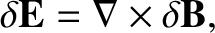(3.91)

which yields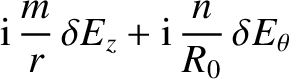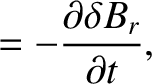(3.92)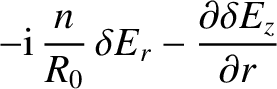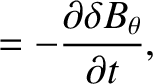(3.93)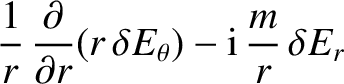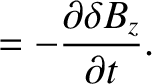(3.94)

Making use of Equations (3.32)–(3.34), as well as the ordering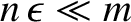, the previous three equations imply that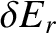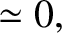(3.95)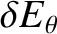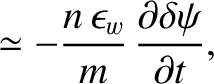(3.96)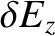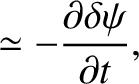(3.97)

where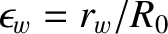. Here, we have assumed that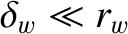, where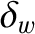is the radial thickness of the wall. We have also made use of the fact that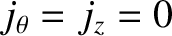outside the plasma.

Inside the wall,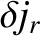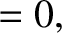(3.98)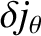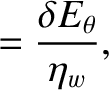(3.99)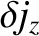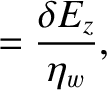(3.100)

where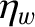is the wall's electrical resistivity. Making use of Equations (3.37), (3.38), (3.96), and (3.97), as well as the fact that, both of the previous two equations reduce to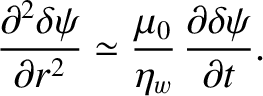(3.101)

Let us adopt the so-called thin-wall limit, according to which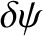is assumed to vary only weakly in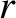across the wall. In this limit, integration of the previous equation across the wall yields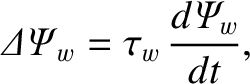(3.102)

where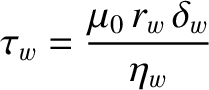(3.103)

is the so-called wall time-constant [4,9]. Here, use has been made of Equations (3.82) and (3.83). The thin-wall limit is valid as long as the wall thickness is less than the resistive skin depth in the wall material. In other words, provided that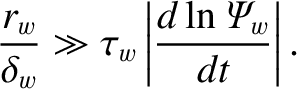(3.104)

In the thin wall limit, Equations (3.82) and (3.95)–(3.100) yield(3.105)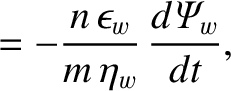(3.106)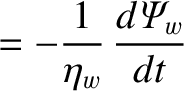(3.107)

inside the wall. Note that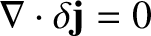, as is required by charge conservation.